Friday, July 29, 2016

(x, why?) Mini: Now Playing . . .

(Click on the comic if you can't see the full image.)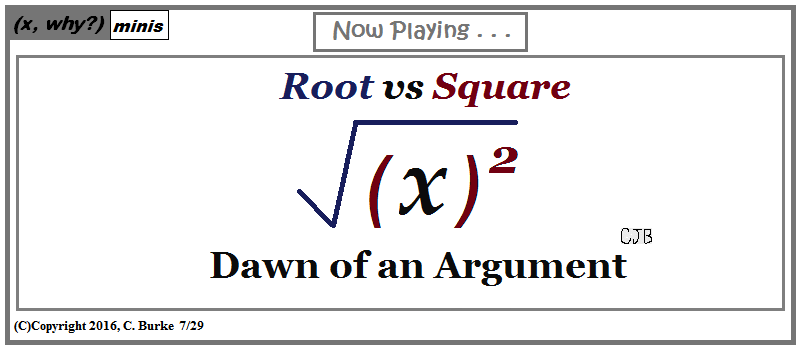They're here to restore Order to your Operations!

But are some operations too powerful?

Come back often for more funny math and geeky comics.Wednesday, July 27, 2016

Daily Regents: Volume and Density (August 2015)

Even though the title says "Daily", I won't be doing them daily any more, until the exams get closer. But they will be frequent.

Geometry, August 2015, Question 25

25. A wooden cube has an edge length of 6 centimeters and a mass of 137.8 grams. Determine the density of the cube, to the nearest thousandth.
State which type of wood the cube is made of, using the density table below.

Density is defined as Mass divided by Volume. (d = m/V). You are given the length of one edge of a cube. The volume of the rectangular prism = length times width times height (V = L * W * H). However, with a cube, all three values are the same, so V = s3.

Density = mass / Volume. Volume of a cube = (edge length)3.
If you substitute the values that we know, we find that Density = mass / (edge)3 = 137.8 / (6)3 = 0.63796..., which rounds to 0.638.

According to the table, the approximate value of Ash wood is 0.638.
The wood is Ash.

Note: Don't forget to identify the wood after finding the density, even if you just circle the correct answer.

Also: If you get "Ash" without showing any work, you will NOT score a point.

Tuesday, July 26, 2016

Daily Regents: Right Triangle Altitude Theorem (June 2015)

Even though the title says "Daily", I won't be doing them daily any more, until the exams get closer. But they will be frequent.

Geometry, June 2015, Question 34

34. In the diagram below, the line of sight from the park ranger station, P, to the lifeguard chair, L, on the beach of a lake is perpendicular to the path joining the campground, C, and the first aid station, F. The campground is 0.25 mile from the lifeguard chair. The straight paths from both the campground and first aid station to the park ranger station are perpendicular.

If the path from the park ranger station to the campground is 0.55 mile, determine and state, to the nearest hundredth of a mile, the distance between the park ranger station and the lifeguard chair.

Gerald believes the distance from the first aid station to the campground is at least 1.5 miles. Is Gerald correct? Justify your answer.

First part: PLC is a right triangle. This means that you can find the missing side using the Pythagorean Theorem.
So (PL)2 + (LC)2 = (LC)2
x2 + .252 = .552
x2 + .0625 = .3025
x2 = .24
x = 0.48989794855 = 0.49 to the nearest hundredth mile.

The second part of the question wants to know, essentially, what is the length of FC. We already know the length of LC, so we need to find the length of FL. We can do this using the Right Triangle Altitude Theorem.
(PL)2 = (FL) * (LC) Remember, we found that x2 = .24. Do NOT square 0.49
.24 = FL * .25
.24 / .25 = FL
FL = .96
FC = FL + LC = .96 + .25 = 1.21
Gerald is incorrect. The distance is shorter than 1.5 miles.

Alternate Solution

All three right triangles in the diagram are similar triangles, so their corresponding sides are proportional.
Therefore, the proportion (base1) / (hypontenuse1) = (base2) / (hypontenuse2) must be a true statement.

Fill in the information we know: .25 / .55 = .55 / FC
Cross-multiply: .25 FC = (.55)2
.25 FC = .3025
FC = .3025 / .25 = 1.21, which is less than 1.5

Wednesday, July 20, 2016

Henways & Wormdoos

(Click on the comic if you can't see the full image.)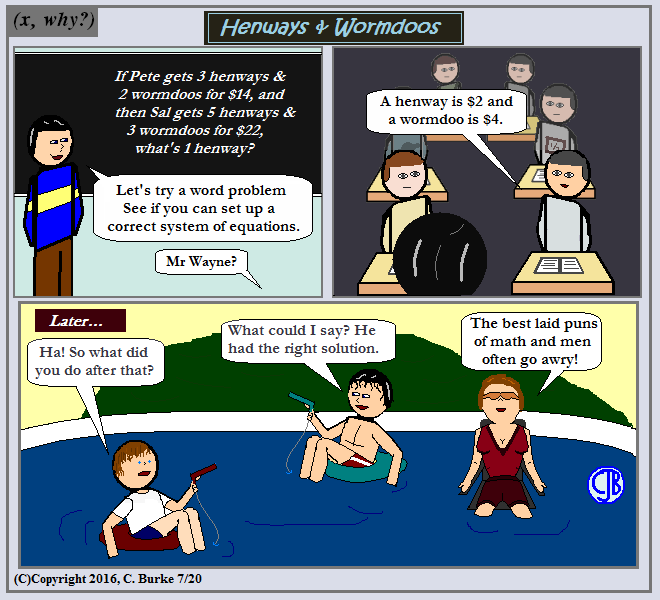Sometimes a good pun is just lost on them. And this math man would prefer to go a-whiskey.

Come back often for more funny math and geeky comics.Monday, July 18, 2016

Polygon Gone!

(Click on the comic if you can't see the full image.)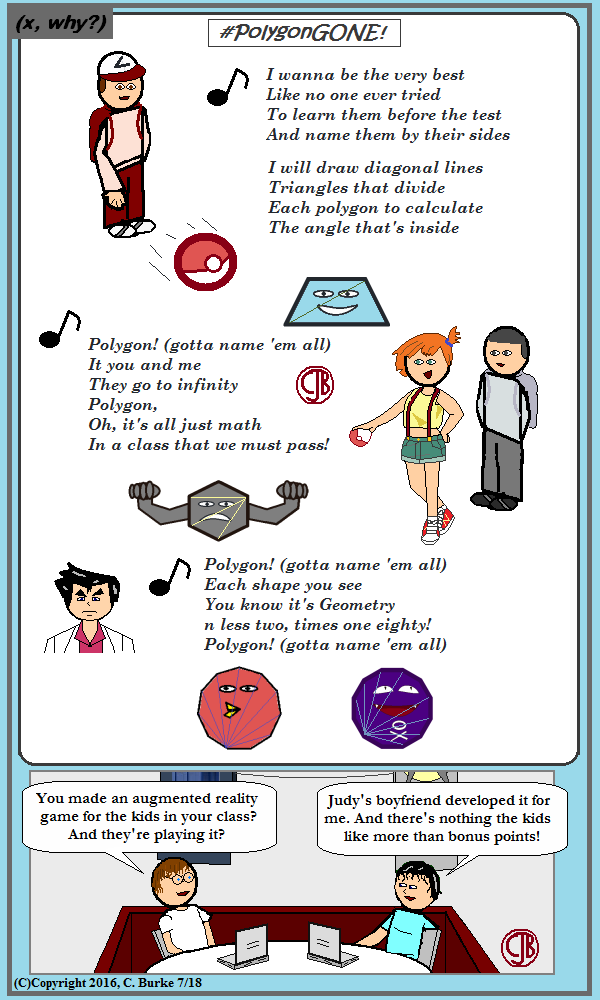Trapezoid! I choose you!

Okay, let's get the math out of the way first.

Polygons: A closed shape, consisting of straight lines that don't overlap each other. They can be concave or convex. Regular polygons have all sides congruent, and all angles congruent. Thus, regular polygons must be convex, with interior angles less than 180 degrees.

You might want to avoid this Definition: Polygon. Or you might want to read the comments for your own amusement.

As mentioned in the comic above, if you draw all the diagonals from one point, you divide the polygon into triangles, each with 180 degrees, and the sum of those is the sum of the interior angles. There will always be two fewer triangles than there are sides, which is the source of the n less two line in the song. Note that a triangle has no diagonals and is composed of a single triangle of 180 degrees, the trivial case for this rule.

In case anyone reading this is new to this blog or my webcomic (did you come from a #PokemonGO or #TMC16 hashtag?), I did want to explain a little about the characters above. They are NOT supposed to be the characters from the show. They are semi-occurring characters made up to imitate the characters in the show ... a little ... sort of. Except for Melissa (aka Missy), she's new. I won't comment on Mr. Wayne's anime-styled hairdo.

The two in the bottom panel, as regular readers know, are math teachers who regularly appear in the strip, but it's summer break, so they're in the yard, having just been in the pool.

For the long-time readers who think they missed something: Judy is the other English teacher who is sometimes seen with Michele. Her boyfriend is Chuck, who appeared a few times in the early years. He works in an office with Frank, aka "Uncle Frank", who has actually appeared more often -- generally, any time I need someone old and tired to express something. Frank, a leap baby, was introduced as being 40, but he's apparently a bit older.

One of these days, I have to do a character map, or at least update a wiki page somewhere.

As for the Polygons in the picture: only two are supposed to be something. The beaked one on the bottom came from comic #957.

I wanted to include a Pentagon with an army theme, but I've done that before. (In actuality, it just wasn't going to fit. You understand, right?)

Finally, I was going to have one of the kids catch a Polygon in a Polyball, and show the figure (the above-mentioned Pentagon) inscribed inside the circle. But I couldn't fit "inscribe" or "circumscribe" in the song! There aren't a lot of good rhymes.

While I'm at it, there aren't a whole lot of useful rhymes for shape either!

Forgive the long missive. I'm not usually this wordy. If you're new here, click on the "Comic" tag to see more. Or just explore. Thanks for stopping by.

Come back often for more funny math and geeky comics.Friday, July 15, 2016

Information ...

(Click on the comic if you can't see the full image.)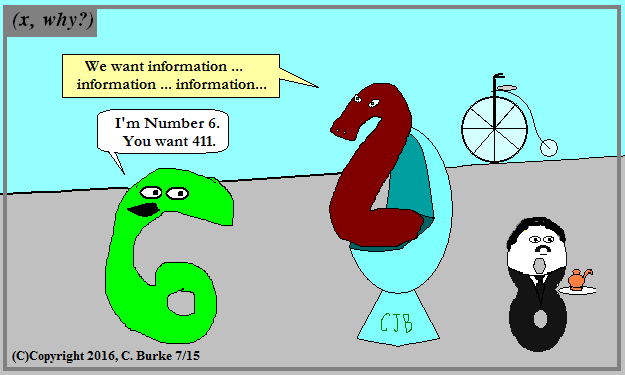Even ''What's the 411?'' is outdated now. Anyone still use it?

This is a leftover joke that I didn't work into the original sequence of strips that started with Six Quits, or The Special Calculator, if you want to know why he quit.

Back then, I didn't run strips consecutively, so there will be some extra strips in between the episodes in the series. Enjoy.

Come back often for more funny math and geeky comics.Thursday, July 14, 2016

Daily Regents: Parallelogram Proof (June 2015)

Even though the title says "Daily", I won't be doing them daily any more, until the exams get closer. But they will be frequent.

Geometry, June 2015, Question 33

33. Given: Quadrilateral ABCD is a parallelogram with diagonals AC and BD intersecting at E.
Prove: Triangle AED = Triangle CEB

You can write a two-column proof or a paragraph proof. I am going to use a paragraph proof to show that they are congruent using SSS. That means that I need to show three sets of sides are congruent. You can also show this using ASA or SAS, but remember to show all three pairs. Don't leave any out.

ABCD is given as a parallelogram. AD is congruent to BC because the opposite sides of a parallelogram are congruent. AC and BD are given as the diagonals of the parallelogram. AC and BD bisect each other because the diagonals of a parallelogram bisect each other. This make AE congruent to CE and BE congruent to DE because of the definition of bisect. Therefore, triangle AED is congruent to triangle CEB because of SSS.

If you wanted to use ASA, you could do so by pointing out that AC and BD are transversals across parallel lines. After that, use Alternate Interior Angles to show EAD = ECB and EDA = EBC.

For SAS, you need a combination of the steps above.

Also, you know that there are vertical angles at point E which are congruent.

Many approaches to a correct answer.

Monday, July 11, 2016

(x, why?) Mini: Long Division

(Click on the comic if you can't see the full image.)Maybe not so much as a conversation as a response to a mini-rant.

I made a note at that point to turn this into a comic. I recently just found that note. Thankfully, I was able to find the original tweets in question.

Many teachers of younger grades are frustrated with long division. It's not easy to teach, and it seems to be an outdated skill since we have calculators. (That said, I've used that skill in Algebra 2 classes with dividing polynomials, which has become more difficult because many of them haven't done long division by hand in five or more years!)

This particular rant (meant to be humorous, from the start) came from Doug Robertson, author of He's the Weird Teacher and The Teaching Text (You're Welcome)

The response was purely me.

For the doubters, here are the tweets in question.

Come back often for more funny math and geeky comics.Wednesday, July 06, 2016

What's Up, Geometry!

(Click on the comic if you can't see the full image.)Shut up and Math with me.

This was the alternative to a similar comic I did last summer. I did the other because it was different. To be honest, I wasn't entirely happy with that final comic, which is probably why this one stayed in my head ever since then.

The only reasons that I didn't do it sooner were because I needed to make new art (Yes, it's similar to This Is Crazy) that fit the song, and had a couple of blank lines. I've reworked these lyrics many times over the past year and yet ... I never wrote the words down!

If I did, it's in a notebook, but it's not on my hard drive or flash drive. Honestly, I thought I'd typed up at least one version so I wouldn't forget it. Also, having it electronically makes it easier to create the text on the comic.

In any case, it's finally done and posted. Hopefully, someone enjoys it enough to comment here or somewhere.

Come back often for more funny math and geeky comics.Monday, July 04, 2016

Happy Fourth of July 2016!

(Click on the comic if you can't see the full image.)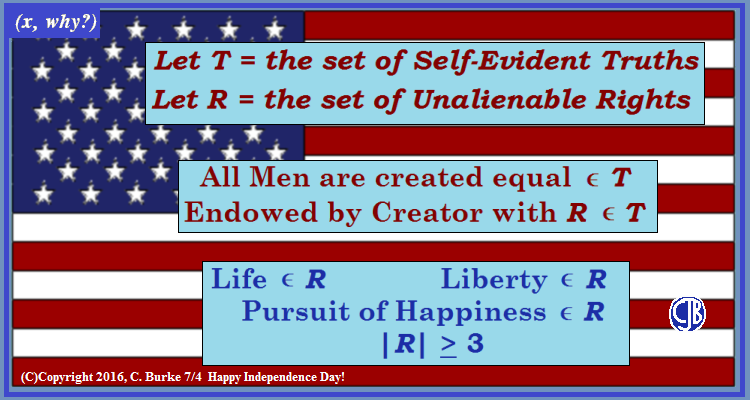There are more elements to T, but the flag is only so big.

The Cardinality of R is at least 3, according to the text "among these are".

Come back often for more funny math and geeky comics.Friday, July 01, 2016

Common Core Geometry, Part 1 (multiple choice), June 2016

What follows is a portion of the Common Core Geometry exam. Illustrations will be added at a later time when they become available.

Part II is posted here.

Part III and IV are posted here.

June 2016 Geometry Regents, Part I

1. A student has a rectangular postcard that he folds in half lengthwise. Next, he rotates it continuously about the folded edge. Which three-dimensional object below is generated by this rotation?

(3). The cylinder. A rectangular postcard folded over is still a rectangle. Roll it along the edge and it becomes a cylinder. (The label of a soup can is a rectangle.)

2. A three-inch line segment is dilated by a scale factor of 6 and centered at its midpoint. What is the length of its image?

(4) 18 inches. If it is centered in the middle, call the left endpoint -1.5 and the right endpoint +1.5. Multiply those by 6 and you get -9 and +9. The distance between those two points is 18.

3. Kevin's work for deriving the equation of a circle is shown below.

x2 + 4x = -(y2 - 20) STEP 1 x2 + 4x = -y2 + 20 STEP 2 x2 + 4x + 4 = -y2 + 20 - 4 STEP 3 (x + 2)2 = -y2 + 20 - 4 STEP 4 (x + 2)2 + y2 = 16
In which step did he make an error in his work?

(2) Step 4. +4 was added to the left, but -4 was added to the right.

4. Which transformation of OA would result in an image parallel to OA?

(1) a translation of two units down. Translations do not affect orientation. The lines will be parallel.

5. Using the information given below, which set of triangles can not be proven similar?

(3). One triangle has sides 2 and 8, the other has 16 and 32. They are not proportional.
Choices (1) and (4) are similar because of SAS. Choice (2) has similarity due to AA.

6. A company is creating an object from a wooden cube with an edge length of 8.5 cm. A right circular cone with a diameter of 8 cm and an altitude of 8 cm will be cut out of the cube. Which expression represents the volume of the remaining wood?

(4) (8.5)3 - (1/3) pi(4)2(8). Volume of a cube is s3. Volume of a cone is (1/3)pi(r)2(h). The diameter is 8, so the radius is 4.

7. Two right triangles must be congruent if

(3) the corresponding legs are congruent. If the legs are congruent with a right angle between them (all right angles are congruent), they are congruent due to SAS. Likewise, if the legs are congruent, then the hypotenuses are congruent, so the triangles are congruent by SSS.

8. Which sequence of transformations will map Triangle ABC onto Triangle A'B'C'?

(4) dilation and rotation. It got bigger, so there's a dilation. Its orientation has changed because of a rotation.

9. In parallelogram ABCD, diagonals AC and BD intersect at E. Which statement does not prove parallelogram ABCD is a rhombus?

(1) AC = DB. Congruent diagonals prove that a parallelogram is a rectangle, not that it is a rhombus.

10. In the diagram below of circle 0, OB and OC are radii, and chords AB, BC, and AC are drawn.

Which statement must always be true?

(2) m<BAC = (1/2)m<BOC. The size of the inscribed angle is half of the central angle. There is nothing that says that AB and AC are congruent, so BAC does not have to be isosceles, even if it looks like it in the image.

11. A 20-foot support post leans against a wall, making a 70° angle with the ground. To the nearest tenth of a foot, how far up the wall will the support post reach?

(4) 18.8. The hypotenuse is 20. The opposite is x. The angle is 70°. Opposite and hypotenuse means use sine.
sin(70) = x / 20, so x = 20 * sin(70) = 18.79... = 18.8

12. Line segment NY has endpoints N(-11,5) and Y(5, -7). What is the equation of the perpendicular bisector of NY?

(1) y + 1 = (4/3)(x + 3). The slope of the line NV is (-7 - 5) / (5 + 11) = -3/4. That means that a line perpendicular to this one has a slope of 4/3. This eliminates choices (2) and (4).
The midpoint is ( (-11+5)/2, (5-7)/2 ) = (-3, -1). Flip the signs to positive, and you get choice (1).

13. In Triangle RST shown below, altitude SU is drawn to RT at U.

If SU = h, UT = 12, and RT = 42, which value of h will make Triangle RST a right triangle with LRST as a right angle?

(2) 6*SQRT(10). (6 times radical 10). Notice that they give RT and not RU. RU = 42 - 12 = 30.
h2 = (30)(12) = 360
h = Sqrt(360) = Sqrt(36)*Sqrt(10) = 6*Sqrt(10).

14. In the diagram below, Triangle ABC has vertices A(4,5), B(2,l), and C(7,3).

What is the slope of the altitude drawn from A to BC?

(4) -5/2. The altitude from A to BC has a slope that is the inverse reciprocal of the slope of BC, because the altitude is perpendicular to BC. The slope of BC is 2/5 (up 2 boxes and right 5). The inverse reciprocal is -5/2.
Note that (-5/2)(2/5) = -1, which is true for inverse reciprocals.

15. In the diagram below, Triangle ERM ~ Triangle JTM.

Which statement is always true?

(4) tan E = TM/JM. Angle E corresponds to angle J. Tan J = opposite / adjacent = TM / JM.

16. On the set of axes below, rectangle ABCD can be proven congruent to rectangle KLMN using which transformation?

(3) reflection over the x-axis.

17. In the diagram below, DB and AF intersect at point C, and AD and FBE are drawn. If AC= 6, DC= 4, FC = 15, m<D = 65°, and m<CBE = ll5°, what is the length of CB?

(1) 10. If CDE = 115, then CDF = 65°, so the triangles are similar. (The vertical angles are congruent also.) This makes the corresponding sides proportional.
4 / 6 = x / 15
6x = (4)(15) = 60
x = 10

18. Seawater contains approximately 1.2 ounces of salt per liter on average. How many gallons of seawater, to the nearest tenth of a gallon, would contain 1 pound of salt?

(2) 3.5. Conversion: 1 pound = 16 ounces. 1 liter = 0.264 gallon. (This comes from the reference table in the back of the book.) Compare ounces to gallons with ounces to gallons.
1.2 / .264 = 16 / x
1.2x = (.264)(16) = 4.224
x = 3.52 = 3.5

19. Line segment EA is the perpendicular bisector of ZT, and ZE and TE are drawn.

Which conclusion can not be proven?

(2) Triangle EZT is equilateral. EZT is isosceles, but not necessarily equilateral.

20. A hemispherical water tank has an inside diameter of 10 feet. If water has a density of 62.4 pounds per cubic foot, what is the weight of the water in a full tank, to the nearest pound?

(1) 16,336/ Density equals mass divided by Volume, in this case pounds per cubic foot. So mass(weight) = Density * Volume.
Volume = (1/2) * (4/3) * pi * r3 = (4 / 6) * pi * 53 = 261.799...
Mass = 62.4 * 261.799 = 16336.2576 = 16,336 pounds.

21. In the diagram of Triangle ABC, points D and E are on AB and CB, respectively, such that AC || DE.

If AD = 24, DB = 12, and DE = 4, what is the length of AC ?

(2) 12. The triangles are similar, so DB/DE = AB/AC. AB = AD + DB = 24 + 12 = 36.
36 / x = 12 / 4
12x = 144
x = 12

22. Triangle RST is graphed on the set of axes below.

(3) 45. The area of a triangles is 1/2*b*h. The base and height are perpendicular to each other. It is easy to see that angle S is a right angle by comparing the slopes. SR has a slope of 2 (you can see this by counting boxes). ST has a slope of -1/2. Multiply 2*(-1/2) = -1, so the lines are perpendicular, and RST is a right triangle.

The length of RS = SQRT(32 + 62) and the length of ST is SQRT(122 + 62).
Multiply 1/2 * SQRT(9 + 36) * Sqrt(144 + 36) = 45. (If you got 90, you forgot the 1/2.)

23. The graph below shows AB, which is a chord of circle 0. The coordinates of the endpoints of AB are A(3,3) and B(3,-7). The distance from the midpoint of AB to the center of circle 0 is 2 units.

What could be a correct equation for circle O?

(1) (x - 1)2 + (y + 2)2 = 29. The center of the circle must be at (1, -2) or (5, -2). The signs must be flipped in the equation of the circle, so any choice with +1, +5 or -2 can be eliminated. Choices (2) and (3) are out. The diameter has to be longer than the chord, so the radius has to be longer than 5.

24. What is the area of a sector of a circle with a radius of 8 inches and formed by a central angle that measures 60°?

(3) 32 * pi / 3. A = (60 / 360) * pi * (8)22 = 64 * pi / 6 = 32 * pi / 3

End of Part I